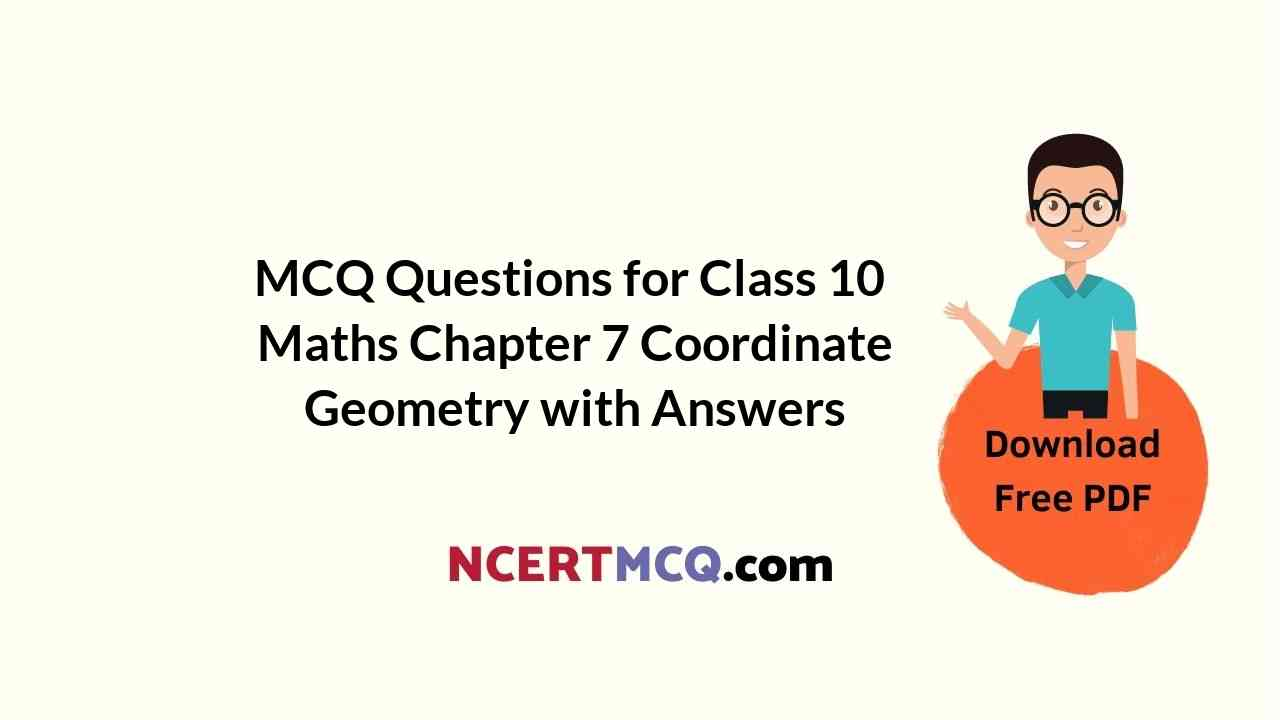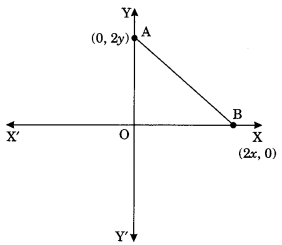Check the below NCERT MCQ Questions for Class 10 Maths Chapter 7 Coordinate Geometry with Answers Pdf free download. MCQ Questions for Class 10 Maths with Answers were prepared based on the latest exam pattern. We have provided Coordinate Geometry Class 10 Maths MCQs Questions with Answers to help students understand the concept very well. https://ncertmcq.com/mcq-questions-for-class-10-maths-with-answers/

Students can also refer to NCERT Solutions for Class 10 Maths Chapter 7 Coordinate Geometry for better exam preparation and score more marks.

## Coordinate Geometry Class 10 MCQs Questions with Answers

Coordinate Geometry Class 10 MCQ Question 1.
The distance of the point P(2, 3) from the x-axis is
(a) 2
(b) 3
(c) 1
(d) 5

Class 10 Coordinate Geometry MCQ Question 2.
The distance between the points A(0, 6) and B(0, -2) is
(a) 6
(b) 8
(c) 4
(d) 2

Coordinate Geometry MCQ Class 10 Question 3.
The distance of the point P(-6, 8) from the origin is
(a) 8
(b) 2√7
(c) 10
(d) 6

MCQ Of Coordinate Geometry Class 10 Question 4.
The distance between the points (0, 5) and (-5, 0) is
(a) 5
(b) 5√2
(c) 2√5
(d) 10

MCQ On Coordinate Geometry For Class 10 With Answers Question 5.
AOBC is a rectangle whose three vertices are A(0, 3), O(0, 0) and B(5, 0). The length of its diagonal is
(a) 5
(b) 3
(c) $$\sqrt{34}$$
(d) 4

Answer: (c) $$\sqrt{34}$$

Class 10 Maths Chapter 7 MCQ Question 6.
The perimeter of a triangle with vertices (0, 4), (0, 0) and (3, 0) is
(a) 5
(b) 12
(c) 11
(d) 7 + √5

Ch 7 Maths Class 10 MCQ Question 7.
The area of a triangle with vertices A(3, 0), B(7, 0) and C(8, 4) is
(a) 14
(b) 28
(c) 8
(d) 6

MCQ Coordinate Geometry Class 10 Question 8.
The points (-4, 0), (4, 0), (0, 3) are the vertices of a
(а) Right triangle
(b) Isosceles triangle
(c) Equilateral triangle
(d) Scalene triangle

Coordinate Geometry MCQ Question 9.
The point which divides the lines segment joining the points (7, -6) and (3, 4) in ratio 1 : 2 internally lies in the

Class 10 Maths Chapter 7 MCQ With Answers Question 10.
The point which lies on the perpendicular bisector of the line segment joining the points A(-2, -5) and B(2, 5) is
(a) (0, 0)
(b) (0, 2)
(c) (2, 0)
(d) (-2, 0)

Chapter 7 Maths Class 10 MCQ Question 11.
The fourth vertex D of a parallelogram ABCD whose three vertices are A(-2, 3), B(6, 7) and C(8, 3) is
(a) (0, 1)
(b) (0, -1)
(c) (-1, 0)
(d)(1, 0)

Class 10 Maths Ch 7 MCQ Question 12.
If the point P(2, 1) lies on the line segment joining points A(4, 2) and B(8, 4), then
(a) AP = $$\frac{1}{3}$$ AB
(b) AP = PB
(c) PB = $$\frac{1}{3}$$ AB
(d) AP = $$\frac{1}{2}$$ AB

Answer: (d) AP = $$\frac{1}{2}$$ AB

MCQ Questions For Class 10 Maths Coordinate Geometry Question 13.
If P ($$\frac{α}{3}$$, 4) is the mid-point of the line segment joining the points Q(-6, 5) and R(-2, 3), then the value of‘a’ is
(a) -4
(b) -12
(c) 12
(d) -6

Class 10 Maths Coordinate Geometry MCQ Question 14.
The perpendicular bisector of the line segment joining the points A(l, 5) and B(4, 6) cuts the y-axis at
(a) (0, 13)
(b) (0, -13)
(c) (0, 12)
(d) (13, 0)

Coordinate Geometry Class 10 MCQs Question 15.
The coordinates of the point which is equidistant from the three vertices of the ΔAOB as shown in the figure.(a) (x, y)
(b) (y, x)
(c) ($$\frac{x}{2}$$, $$\frac{y}{2}$$)
(d) ($$\frac{y}{2}$$, $$\frac{x}{2}$$)

MCQ Questions For Class 10 Maths Chapter 7 Question 16.
A circle drawn with origin as the centre passes through ($$\frac{13}{2}$$, 0). The point which does not lie in the interior of the circle is
(a) (-$$\frac{3}{4}$$, 1)
(b) (2, $$\frac{7}{3}$$)
(c) (5, –$$\frac{1}{2}$$)
(d) (-6, $$\frac{5}{2}$$)

Answer: (d) (-6, $$\frac{5}{2}$$)

MCQ Of Chapter 7 Maths Class 10 Question 17.
A line intersects the y-axis and x-axis at the points P and Q respectively. If (2, -5) is the mid-point of PQ, then the coordinates of P and Q are respectively
(a) (0, -5) and (2, 0)
(b) (0, 10) and (-4, 0)
(c) (0, 4) and (-10, 0)
(d) (0, -10) and (4, 0)

Answer: (d) (0, -10) and (4, 0)

Coordinate Geometry Class 10 MCQ Pdf Question 18.
The area of a triangle with vertices (a, b + c), (b, c + a) and (c, a + b) is
(a) (a + b + c)²
(b) 0
(c) a + b + c
(d) abc

MCQs On Coordinate Geometry Class 10 Question 19.
If the distance between the points (4, P) and (1, 0) is 5, then the value of P is
(a) 4 only
(b) ± 4
(c) -4 only
(d) 0

Coordinate Geometry Class 10 MCQ With Answers Question 20.
If the points A(1, 2), O(0, 0), C(a, b) are collinear, then
(a) a = b
(b) a = 2b
(c) 2a = b
(d) a = -b